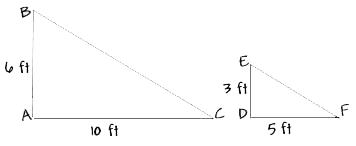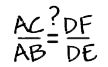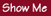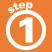Home    |    Teacher    |    Parents    |    Glossary    |    About UsTwo figures that have the same shape are said to be similar.

When two figures are similar, the ratios of the lengths of their corresponding sides are equal.

To determine if the triangles below are similar, compare their corresponding sides.Are these ratios equal?Homework Help | Pre-Algebra | Ratios and ProportionsEmail this page to a friendSearch·  Ratios·  Proportions·  Distance, rate     and time·  Similar figuresFirst Glance In Depth Examples WorkoutSimilar figures